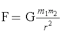Often called Newton’s 4th law, this is actually the Law of Universal Gravitation.   It is Newton’s explanation of that force that we call gravity.

The law states that every object (with mass) in the universe attracts every other object in the universe with a force (gravity) that is directly proportional to the product of their masses and inversely proportional to the square of the distance between their centers. This is one of the inverse-square laws.

in formula form,where F = force of gravity between the objects (m1 and m2)

G = universal gravitation constant = 6.67 X 10-11 Nm2/kg2

m1 = 1st object

m2 = 2nd object

r = distance between the center of the two objects

The diagram below illustrates what is going on with the law. Be careful when asked about the force on m1 versus the force on m2….they are always equal because it is the force between them.Refer back to Newton’s 2nd law of motion. Since gravity is frequently an unbalanced force (i.e., when an object is dropped or thrown through the air), it produces an acceleration. This is called the acceleration due to gravity (g) and for all practical purposes, is a constant on or near the Earth’s surface.

g = 9.8 m/s2 = 980 cm/s2 = 32 ft/s2

Another key concept, the acceleration due to gravity is the same for all objects, regardless of their mass. No, this does not mean that all objects weigh the same. It means that they will be pulled down and will accelerate at the same rate. (Note: the exceptions are due to air resistance.)

The effects of gravity on Earth’s inhabitants are quite varied.

(source)